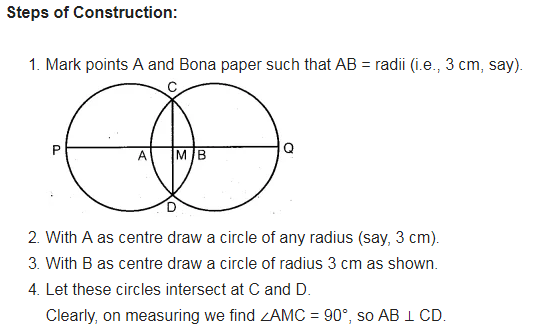# Let A, B be the centres of two circles of equal radii; draw them so that each one of them passes

Let A, B be the centres of two circles of equal radii; draw them so that each one of them passes through the centre of the other. Let them intersect at C and D.
Examine whether AB and CD are at right angles.